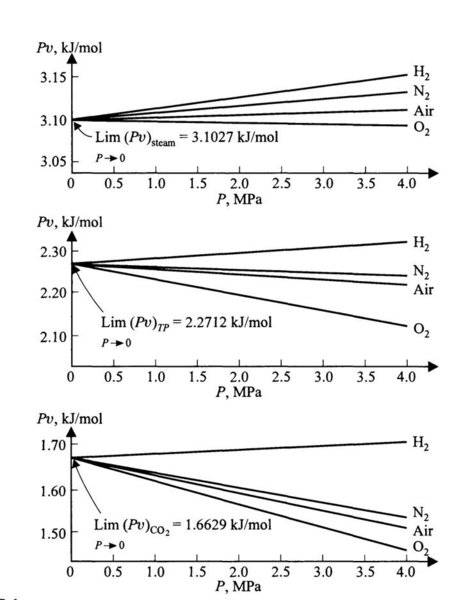# Experimental Virial Equation for real gases

• B
• tanaygupta2000

#### tanaygupta2000

In the experimental virial equation for real gases,
Pv = A(1+BP) (for small values of P)
as 'P' approaches zero, then why do we get some finite value of 'Pv' instead of approaching to zero value, since 'Pv' is directly proportional to 'P' ?

{P = Pressure of gas, v = Molar volume of gas, A,B = Virial Constants}#### Attachments

At low pressure, gases behave more and more ideally. Boyle's law has ##PV = ## constant. A non-zero constant ! ##PV = n RT## in the ideal gas law .
At higher pressures you get deviations and the virial expansion is one way to introduce a correction.

Read up in e.g. Smith & van Ness: Chemical engineering thermodynamics

PS has your source some caption with the three figures ? What do they represent ?